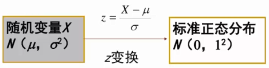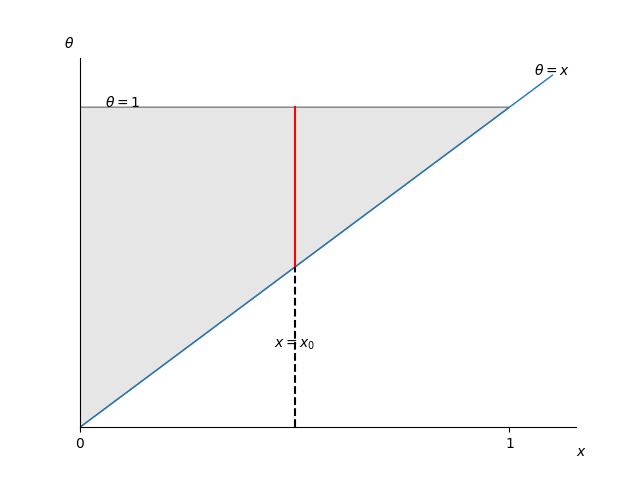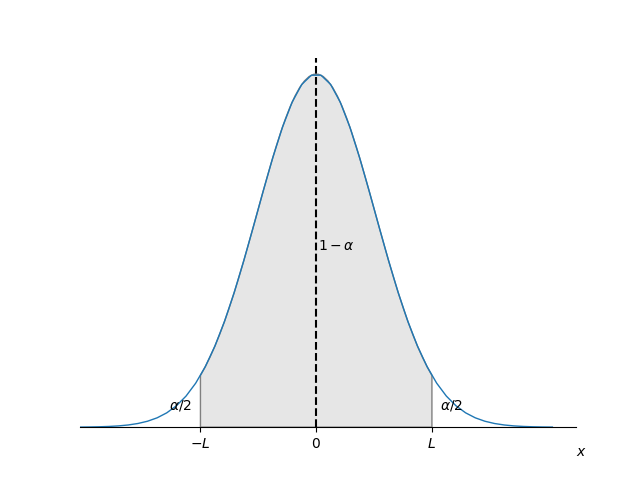• 统计推断 抽样误差与标准误 抽样误差：由于个体差异产生的，抽样造成的样本统计量与总体参数之间的差别，由于抽样方法、个体差异导致 标准误：表示样本统计量抽样误差大小的统计量 总体标准误=总体标准差/总体数开...
统计推断
抽样误差与标准误
抽样误差：由于个体差异产生的，抽样造成的样本统计量与总体参数之间的差别，由于抽样方法、个体差异导致
标准误：表示样本统计量抽样误差大小的统计量

总体标准误=总体标准差/总体数开平方
${\sigma}_{\bar{x}}=\frac{\sigma}{\sqrt{n}}$
样本标准误=样本标准差/样本数开平方
$S_{\bar{x}}=\frac{S}{\sqrt{n}}$

标准差与标准误的区别

指标
意义
应用

标准差
衡量离散程度
比如描述正态分布的频数分布

标准误
衡量抽样误差大小
比如总体均数区间估计

t分布
t分布只有一个参数，自由度v。
t分布特征：

单峰分布，以0为中心，左右对称
自由度v越小，则t值越分散，峰值越矮而尾部越翘
自由度v越大，越接近正态分布

t值等于均值减去总体标准差然后再除以样本标准误，v表示自由度。
$t=\frac{\bar{X}-\mu}{S_{\bar{x}}}=\frac{\bar{X}-\mu}{\frac{S}{\sqrt{n}}},v=n-1$

如果样本均数$\bar{X}$服从总体均数$\mu$、总体标准差为$\sigma_{\bar{x}}$的正态分布$N(\mu,\sigma_{\bar{X}}^2)$，则通过Z值转换为标准正态分布$N(\mu,1^2)$，即z分布；
实际中，由于总体标准差为$\sigma_{\bar{x}}$经常是未知的，所以一般用样本标准差$S_{\bar{X}}$代替，则转换后的分布不再服从标准正态分布，而是服从t分布。

z值标准化用于将t分布转换为z分布，z值等于每个数据减去均值再除以标准差

理论：
$z=\frac{X-\mu}{\sigma}$
实际：
$z=\frac{X-\bar{X}}{S}$

用于判断哪些数值为异常值，z值大于3为异常值，大于5为极端值。参数估计
用样本统计量推断总体参数

点估计：用相应样本统计量直接作为总体参数的估计值
区间估计：按预先给定的概率所确定的包含未知总体参数的一个范围。
$\bar{X}-t_{\alpha/2,v}S_{\bar{X}}<\mu<\bar{X}+t_{\alpha/2,v}S_{\bar{X}}$

置信度：

t值
置信度

1.65
90%

1.98
95%

2.38
99%

假设检验
基本思想
基本思想是小概率反证法，从问题对立面（H0）出发，间接判断要解决的问题（H1）是否成立，然后在H0成立的条件下计算检验统计量，最后得到P值来判断。当P值小于预先设定的显著性水平$\alpha$时，就属于小概率事件，根据小概率事件原理——小概率事件在一次抽样中发生的可能性很小，如果发生了，则有理由怀疑原假设H0，认为其对立面H1是成立的。
步骤

建立检验假设（H0，H1），确定显著性水平
$\alpha$一般取5%
H0通常定义为两个变量之间不存在相关性或者没有显著差异
选择合适的检验方法，计算统计量
根据变量类型，统计推断的目的，是否满足特定条件等，选择相应的校验统计量（t值、F值、Z值、卡方值）
计算P值，与显著性水平对比，
$p<\alpha$，拒绝原假设H0，则接受研究假设H1
$p>\alpha$，接受原假设H0，则拒绝研究假设H1

第一类错误、第二类错误

第一类错误（弃真错误）：原假设H0正确，而检验结果把它否定了
第二类错误（取伪错误）：原假设H0不正确，而检验结果把它肯定了
显著性水平$\alpha$：犯第一类错误的最大概率


展开全文• 统计推断-经典统计推断基本问题统计学与概率论贝叶斯统计与经典统计推断模型与推断变量术语解释经典参数估计术语最大似然估计均值和方差的估计置信区间求近似的置信区间基于方差近似估计量的置信区间线性回归最小...
统计推断-经典统计推断基本问题统计学与概率论贝叶斯统计与经典统计推断模型与推断变量术语解释经典参数估计术语最大似然估计均值和方差的估计置信区间求近似的置信区间基于方差近似估计量的置信区间线性回归最小二乘法合理性贝叶斯线性回归多元线性回归非线性回归线性规划注意事项简单假设检验内曼-皮尔逊引理显著性检验广义似然比和拟合优度检验
基本问题

统计推断是什么？
统计推断是从观测数据推断未知变量或未知模型的有关信息的过程。
统计推断的用途是什么？
统计推断可用于“参数估计”,“假设检验”，“显著性检验”
统计推断的研究思路是什么?
主要有两种思路:“贝叶斯统计推断” 和“经典统计推断”。(大局方法)
统计推断具体使用的"算法"有哪些？
最大后验概率准则,最小均方估计，最大似然估计，回归，似然比检验等。(小方法)

统计学与概率论
“统计学”与“概率论”在认识论上有明显的区别。
概率论是建立在概率公理上的系统自我完善的数学课题。我们会假设一个完整的特定的概率模型满足概率公理，然后用数学方法研究模型的一些性质。概率模型无需与现实世界相一致，它值对概率公理负责。
统计学是针对一个具体的问题，寻求合理的研究方法，希望得到合理的结论。这就存在很大的自由度，采取不同的研究方法，结论可能不同。通常我们会附加一些限制条件，以便得到“理想结论”。

正是由于统计学的这种特征，现实社会存在许多人为制造的"理想结论"，这些结论可能来源于真实的数据，但研究方法是人为选定的。

贝叶斯统计与经典统计
贝叶斯统计与经典统计(频率学派)是两种突出但对立的思想学派。
最重要的区别就是如何看待未知模型或变量。贝叶斯学派将其看成已知分布的随机变量。而经典统计将其看成未知的待估计的量。
贝叶斯方法将统计拉回“概率论”的研究领域，使得每个问题只有一个答案。经典统计将未知量看作一种参数，它是一个常数，未知需要估计。
从现实角度来看，贝叶斯统计主张将假设的先验分布公开，即研究过程公开了。贝叶斯统计推断涉及到多维度积分，计算困难，所以贝叶斯学派的最新成功可能集中于如何计算上。
推断模型与推断变量
这两种问题有细微的区别。推断模型是为了研究某种现象或过程的一般规律，以期能够预测未来现象的结果。推断变量是从已知的量，推测未知的量，例如从gps信息推断所处于的位置。
术语解释

参数估计:对参数进行估计，使得在某种概率意义下估计接近真实值。
假设检验:未知参数根据对立的假设可能取有限个值，选择一个假设，目标是使犯错误的概率最小。
显著性检验：对于一个给定的假设，希望发生错误(“接受错误”与“拒绝正确”)的概率适当地小.
最大似然估计:在选择参数$\theta$时，使得观测数据最有可能出现，即观测到当前数据的概率达到最大。
线性回归：对于给定的一组观测数据，采用线性拟合的方式建立模型。约束条件是使观测数据与模型值的差的平方和最小。(最小二乘法)
似然比检验:对于给定的两个假设，根据他们发生的可能性的比值选择其中一个，使得犯错的概率适当小。

经典参数估计
虽然把$\theta$当作常数，而不是随机变量，但仍然把$\theta$估计量当作随机变量$\hat\Theta$，因为$\hat\theta$一般而言是$x$的函数,$\hat\theta=g(x)$，所以也有:$\hat\Theta=g(X)$。也可以写成$\hat\Theta=g(X;\theta)$，这个式子的意思是$\hat\Theta$是$\theta$的数值函数。
术语
$\hat\Theta_n$是未知参数$\theta$的估计量，也即$n$个观测$X_1,X_2,...,X_n$($X$的分布依赖于$\theta$)的函数:

估计误差:$\tilde \Theta_n=\hat\Theta_n-\theta$
估计量偏差:$b_\theta(\hat\Theta_n)=E(\hat\Theta)-\theta$
估计量的偏差，方差，期望是\theta的函数，而估计误差是$(X_1,X_2,....,X_n,\theta)$的函数
无偏估计的定义:如果$E(\hat\Theta)=\theta$对$\theta$所有可能的取值都成立
渐进无偏的定义:如果$\lim _{n\rightarrow \infty}{E(\hat\Theta_n)}=\theta$.
称$\hat\Theta$是$\theta$的相合估计序列，如果对于所有的$\theta$可能的取值，$\hat\Theta$依概率收敛到参数$\theta$的真值:$\forall \epsilon >0,\lim _{n\rightarrow \infty}{P(|\hat\Theta - \theta|>\epsilon)=0.}$
$E(\tilde\Theta ^2)=E[(\hat\Theta_n-\theta)^2]=var(\hat\Theta_n-\theta)+E^2(\hat\Theta_n-\theta)=var(\hat\Theta_n)+b^2 _\theta(\hat\Theta)$,这个式子建立了估计均方误差、估计量方差、估计偏差的关系。可以看出均方误差也是$\theta$的函数。如果均方误差不变，则减小方差会增大偏差，减小偏差会增大方差。

最大似然估计
定义:设观测向量$X=(X_1,X_2,...,X_n)$的联合分布列为$p_{X_1,X_2,..,X_n}(x_1,x_2,...,x_n;\theta)=p_{X}(x_1,x_2,...,x_n;\theta)$,最大似然估计就是寻求参数$\theta=\hat\theta$使得关于$\theta$的函数$p_{X}(x_1,x_2,...,x_n;\theta)$达到最大,即寻求参数$\theta=\hat\theta$使得观测值$X$最有可能出现。
当$X$为连续随机变量时,$p_X$用概率密度函数$f_X(x_1,x_2,...,x_n;\theta)$代替。
如果$X_1,X_2,...,X_n$是相互独立的，那么$p_X=p_{X_1}p_{X_2}...p_{X_n},$此时可用对数似然函数来简化计算:$ln(p_X)=lnp_{X_1}+...+lnp_{X_n}$

与贝叶斯最大后验概率准则对比:
最大后验概率准则:求$\theta=\hat\theta$使得$p_\Theta( \theta)p_{X|\Theta}(x|\theta)$取最大值。
最大似然估计:求$\theta=\hat\theta$使得$p_{X}(X;\theta)$取最大值。
可以看出当$\Theta$是均匀分布时,最大后验准则等价于最大似然估计。均匀分布即$\Theta$取任何值的概率都相等，这就是经典统计推断与贝叶斯统计推断的不同之处。

如果$\theta$的最大似然估计是$\hat\theta$，那么$g(\theta)$的最大似然估计是$g(\hat\theta)$.这里要求$g(x)$是一一映射函数。

举例:某人上班迟到时间是一个随机变量X,服从参数为$[0,\theta]$上的均匀分布,$\theta$未知，是随机变量$\Theta$的一个值，$\Theta$服从$[0,1]$上的均匀分布。假设某次迟到时间为x。用最大似然估计来估计$\theta$。
流程:
$f_X(x;\theta)=\frac{1} {\theta}$
画出$\theta-x$的取值范围图:$\theta$的取值范围图中阴影部分。对于观测值$x=x_0,\theta$的取值范围为图中红线部分。显然当$\theta =x$时能使$f_X$达到最大。所以$\theta$的最大似然估计$\hat\Theta=X$.

均值和方差的估计
利用经典统计推断一个概率分布的均值和方差(不一定是“最大似然估计”)。
这里的目标是通过样本推断总体的无偏估计均值和方差。
假设条件:

观测向量$(X_1,X_2,...,X_n)$是独立同分布
均值为未知参数$\theta$.方差为未知参数$v=\sigma^2$
对于均值最自然的估计量是样本均值:
$\hat\Theta=M_n=\frac{X_1+X_2+...+X_n}{n}$
样本均值当作均值估计量时有:

$E(M_n)=\theta$,所以$M_n$是$\theta$的无偏估计量。
$E(X_iM_n)=\theta^2=E(X_i)E(M_n)$，所以$M_n$和$X_i$不相关。
$var(M_n)=var(X_1+X_2+...+X_n)/n^2=v/n$.可见方差和均方不依赖
均方误差$E[(\hat\Theta-\theta)^2]=E[(M_n-\theta)^2]=E[(M_n-E(M_n))^2]=var(M_n)$,对于无偏估计量总有方差等于均方误差。上式也说明估计量$M_n$的方差和均方误差都不依赖于$\theta$(不是所有的估计量都有这个性质).
样本均值$M_n$不一定是方差最小的估计量。例如取$\hat\Theta=0$，此时方差是0，由于“均方误差=方差+偏差的平方”，此时偏差不是0，均方误差也依赖于$\theta$了。

对于方差$v$的估计量最自然的选择:
$\hat V=\frac{1}{n}\sum _{i=1}^{n}(X_i-M_n)^2=\overline S_n^2$
那么$\overline S_n^2$是否是$v$的无偏估计量呢？
$E(\hat V)=E(\overline S_n^2)=\frac{1}{n} \sum_{i=1}^{n}[E(X_i^2)-2E(X_iM_n)+E(M_n^2)]\\=\frac{1}{n}[n(v+\theta^2)-v-n\theta^2]\\=v-\frac{v}{n}=\frac{n-1}{n}v$
说明$\overline S^2$不是$v$的无偏估计量，比方差$v$少$v/n$，但$\overline S^2$是渐进无偏的.为了得到$v$的无偏估计量，可以对$\overline S^2$进行一定的缩放:
$E(\hat S_n^2)=E[\frac{n}{n-1}*\overline S_n^2]=v$
所以方差的估计量有两个:
$\overline S^2=\frac{1}{n}\sum _{i=1}^{n}(X_i-M_n)^2,\hat S^2=\frac{1}{n-1}\sum _{i=1}^{n}(X_i-M_n)^2$

无偏估计方差为什么会出现有(n-1)?
方差的计算式子中$E[(X-\mu)^2]$中$\mu$是常数，方差为0.而在这里的估计过程中,期望和方差都是待估计量，都不是常数。所以样本的方差$\overline S_n^2$包含了样本均值的方差$v/n$和样本的无偏方差$\hat S_n^2$.

置信区间
粗略地说，置信区间的作用是使用"区间估计"代替“点估计”,使得"区间"包含真值的概率达到适当的水平。这个适当的水平即"置信水平",通常设为$1-\alpha$.置信区间设为$[\hat\Theta_n^-,\hat\Theta_n^+]$,要求置信区间包含真值的概率达到置信水平:
$P(\hat\Theta_n^- \le \theta \le \hat\Theta_n^+)\ge (1-\alpha)$
称$[\hat\Theta_n^-,\hat\Theta_n^+]$为$(1-\alpha)$置信区间。

请注意，这里随机变量是与区间相关的。例如假设$[0,1]$是$\theta$的$0.95$置信区间，准确的理解是$[0,1]$包含$\theta$的概率是$0.95$，而不能说$\theta$落在$[0,1]$内的概率是$0.95$.

求近似的置信区间
在很多重要的模型中$\hat\Theta$的分布是渐进正态无偏的(中心极限定理)，在$n\rightarrow \infty$时,$E(\hat\Theta) \rightarrow \theta$,所以:
$Z_n=\frac{\hat\Theta_n - \theta}{\sqrt{var(\hat\Theta)}}$
服从标准正态分布.
查表$\Phi(1.96)=P(Z_n\le1.96)=0.975$
假设$\theta$处于置信区间的中点，那么$\hat\Theta^-=\hat\Theta-l,\hat\Theta^+=\hat\Theta+l$,于是有：
$P(-l \le \hat\Theta-\theta \le +l)\ge (1-\alpha)$
$(\hat\Theta-\theta)$正态分布的对称轴是0(因为均值为0).如图阴影部分面积为$(1-\alpha)$,那么就应该有$\Phi(L)=1-\alpha/2$
如果$\alpha =0.05$,置信水平是$0.95$,查表$\Phi(1.96)=1-0.25=0.975$,
所以$L=1.96=\frac{\hat\Theta-\theta}{\sqrt{var(\hat\Theta)}}$,
$\hat\Theta - L\sqrt{var(\hat\Theta)} \le \theta \le \hat\Theta + L\sqrt{var(\hat\Theta)}$
其中$\Phi(L)=1-\alpha/2$.上式就是$(1-\alpha)$置信水平的置信区间。

假设$\theta$是固定的，运用相同的统计过程建立了n个$0.95$置信区间。可以预期在n个置信区间中，将有95%的置信区间包含$\theta$.

基于方差近似估计量的置信区间
在上面的置信区间式子中包含估计量的方差$var(\hat\Theta)$,如果用样本均值$\hat\Theta=M_n=\frac{X_1+X_2+...+X_n}{n}$
来估计$\theta$,用无偏估计量:
$\hat S^2=\frac{1}{n-1}\sum _{i=1}^{n}[(X_i-\theta)^2]$
来估计方差.那么就可以用$\hat S^2/n$来估计$var(\hat\Theta)=var(M_n)=v/n$。
对于给定的$\alpha$,可以构造一个近似的$(1-\alpha)$的置信区间,即:
$[\hat\Theta-L\frac{\hat S}{\sqrt n},\hat\Theta+L\frac{\hat S}{\sqrt n}]$,
其中$\Phi(L)=1-\alpha/2$.
整个过程有两个近似:

将$\hat\Theta$看作正态分布的随机变量
用估计$\hat S^2/n$来代替来$\hat\Theta$真实的方差$var(\hat\Theta)$

所以这里实际上是用正态分布去近似了一个不是正态分布的概率。为了$\Phi(L)=1-\alpha/2$更精确,用一个比正态分布更好的$t$-分布去计算$L$.
现在定义一个随机变量:
$T_n=\frac{\hat\Theta}{\hat S_n /\sqrt n}$
,称$T_n$为自由度$n-1$的$t$-分布。
此时$L$的计算式子为:
$\Psi_{n-1}(L)=1-\alpha/2$.
其中$\Psi_{n-1}(z)$是自由度为$n-1$的t-分布的概率分布函数.
由t-分布和正态分布的关系,可以得出t-分布应该和正态分布函数的图像近似。

举例:利用电子天平得到一个物体重量的八次测量,观测值是真实的质量加上一个随机误差，随机误差服从$(0,v)$的正态分布,假设每次观测误差都是相互独立的，观测值如下:
$X=(0.5547,0.5404,0.6364,0.6438,0.4917,0.5674,0.5664,0.6066)$
计算95%置信区间。
这类不知道方差的情况，使用t-分布来近似计算置信区间.
流程:

计算均值和方差.
$\theta=E(\hat \Theta)=E(M_n)=0.574$,方差的估计是$\frac{\hat S^2}{n}=\frac{1}{n-1}\sum _{i=1}^{n}[(X_i-\theta)^2]=3.2952*10^{-4}$
因而标准差估计为:$\sqrt{3.2952*10^{-4}}=0.0182$
查t-分布表
查表使得:$\Psi_{7}(L)=1-\alpha/2=0.975=\Psi(2.365)$
计算置信区间$[\hat\Theta-L\frac{\hat S}{\sqrt n},\hat\Theta+L\frac{\hat S}{\sqrt n}]$
置信区间为:$[\hat\Theta-0.043,\hat\Theta+0.043]$.使用样本均值作为$\hat\Theta$的估计则$0.95$置信区间为:$[0.531,0.617]$

方差的估计方式可以有多种，所以答案不是唯一的，这里采用的是样本的无偏估计方差$\hat S^2$。

线性回归
线性回归的典型应用:已知$n$组数据对$(x_i,y_i)$,使用线性回归$y=cx+d$来拟合$x,y$之间的关系。
用最小二乘法推导计算公式:
$\begin{cases} cx_1+d=y_1 \\ cx_2+d=y_2 \\ ... \\ cx_n+d=y_n \end{cases}$
将此式写成矩阵形式$Az=b$:
$A=\begin{pmatrix}x_{1} & 1 \\x_2 & 1 \\... & ... \\x_n & 1\end{pmatrix}, z=\begin{pmatrix} c \\ d \end{pmatrix}, b=\begin{pmatrix} y1 \\ y2 \\...\\y_n \end{pmatrix}$
$Az=b,A^TAz=A^Tb$
$z=(A^TA)^{-1}A^Tb$
计算:
$A^T=\begin{pmatrix} x_1 & x_2 & ... & x_n \\ 1 & 1 & ... & 1 \end{pmatrix}$
$A^TA=\begin{pmatrix} \sum x_i^2 & \sum x_i \\ \sum x_i & n \end{pmatrix}$
由于:$\begin{pmatrix} a & b \\ c& d \end{pmatrix}^{-1}=\frac{1}{ad-bc}\begin{pmatrix} d & -b \\ -c & a \end{pmatrix}$
$(A^TA)^{-1}=\frac{1}{n\sum x_i^2-(\sum x_i)^2}\begin{pmatrix} n & -\sum x_i \\ -\sum x_i & \sum x_i^2 \end{pmatrix}$
$A^Tb=\begin{pmatrix} \sum(x_iy_i) \\ \sum y_i \end{pmatrix}$
$z=(A^TA)^{-1}A^Tb=\frac{1}{n\sum x_i^2-(\sum x_i)^2}\begin{pmatrix} n & -\sum x_i \\ -\sum x_i & \sum x_i^2 \end{pmatrix} \begin{pmatrix} \sum(x_iy_i) \\ \sum y_i \end{pmatrix}$
$=\frac{1}{n\sum x_i^2-(\sum x_i)^2} \begin{pmatrix} n\sum(x_iy_i)-\sum x_i \sum y_i \\ -\sum x_i \sum(x_iy_i)+\sum(x_i^2)\sum y_i \end{pmatrix}$
设$n\overline x=\sum (x_i),n\overline y=\sum y_i$,则有:
$\sum \overline x^2 =1/n*\sum (x_1+x_2+...+x_n)\overline y=\sum x_i\overline x$
$\sum \overline x\overline y=\sum (x_i\overline y)=\sum (\overline x y_i)$
推导一个分母:
$n(x_1^2+x_2^2+...+x_n^2)-(x_1+x_2+...+x_n)^2=n(x_1^2+x_2^2+...+x_n^2)-(n\overline x)^2\\=n[(x_1^2+x_2^2+...+x_n^2)-n\overline x^2]=n\sum (x_i^2-\overline x^2)=n\sum(x_i^2-2x_i\overline x + \overline x^2)\\=n\sum(x_i-\overline x)^2$
类似的，最终可以化称下面这个式子:
$c=\frac{\sum (x_i-\overline x)(y_i-\overline y)}{\sum (x_i-\overline x)^2}$
$d=\overline y - c\overline x$
最小二乘法合理性
这一节是尝试说明最小二乘法的合理性，它同统计理论的许多方法类似。
在贝叶斯线性最小均方估计中,假设估计量和观测向量是线性关系，即:$\hat Y=aX+b$.求得的线性最小均方估计为:
$\hat\Theta=\frac{cov(Y,X)}{\sigma _X^2}(X-\mu_X)+\mu_Y$
即$a=\frac{cov(Y,X)}{\sigma _X^2},b=\mu _Y-a\mu _X$
由于不知道$(X,Y)$的分布，如果用样本均值代替上式中的分布参数:
$\mu _X=\overline x,\mu _Y=\overline y$
$cov(X,Y)=E[(X-\mu _X)(Y-\mu _Y)]=\sum [(x_i-\overline x)(y_i-\overline y)]/n$
$\sigma_X^2=[\sum(x_i-\overline x)^2]/n$
则
$a=\frac {\sum [(x_i-\overline x)(y_i-\overline y)]}{\sum(x_i-\overline x)^2}$
可见最小二乘法和线性最小均方估计是类似的。
贝叶斯线性回归
线性回归是一种方法，用线性关系拟合两个量之间的关系，其特点是观测量到拟合直线的距离的平方和最短。所以无论是经典统计推断还是贝叶斯统计推断，凡是要求这种拟合关系的场合，都可以运用线性回归。
多元线性回归
多元线性回归的公式，比一元复杂得多。结合最小二乘法和矩阵的知识，则相对容易求解。常见思路:$y=a+a_1x_1+a_2x_2+a_3x_3$,先考虑能否求得$x_2=h_2(x_1),x_3=h_3(x_1)$,这样就能使多远线性规划问题化为一元线=线性回归问题。
非线性回归
非线性回归通常没有闭合式解，对于具体问题需要具体的应对方法。
线性规划注意事项
在解决实际问题问题时，线性回归分析需要考虑以下问题:

异方差性。实际问题中观测值的分布的方差可能具有很大的差异性，这样方差很大的观测值对于参数估计将造成不恰当的影响，适当的补救办法是采用加权最小二乘准则。
非线性。实际问题并非近似线性关系，用线性回归处理就不合适量。
多重共线性。如果有真实关系:$y=2x+1,z=x$，那么对于$y=ax+bz+c$,就无法区分$x,z$对$y$的贡献。
过度拟合。用8次多项式拟合8个数据点，显然这是不合适的。经验:数据点的数量应当是待估参数的5～10倍.
线性关系不是因果关系，而只是说明相关性。

简单假设检验
假如未知参数$\theta$只有两种取值$\{\theta_0,\theta_1\}$,假设检验就是判断接受哪一种假设，分别设为$\{H_0,H_1\}$.
现将观测向量$X$的空间分为两类:1.拒绝域$R$：若$X\in R$,则认定$H_0$为假，拒绝；2.接受域$R^c$.

第一类错误:错拒.即$H_0$正确而拒绝.$\alpha(R)=P(X\in R;H_0)$
第二类错误:受假.$H_0$错误而接受。$\beta(R)=P(X\notin R;H_1)$
似然比:两种假设情形下概率的比值():
$L(x)=\frac{p_X(x;H_1)}{p_X(x;H_0)}$
似然比的临界值需要根据问题来适当选取。
举例子说明。

现在想检验一骰子是否六面均匀，给出两个假设:
$H_0$:骰子均匀。$p_X(x;H_0)=1/6$.
$H_1$:骰子不均匀。$p_X(x;H_1)=\begin{cases} 1/4,x=1,2 \\ 1/8,x=3,4,5,6 \end{cases}$
1.先计算似然比函数:
$L(x)=\begin{cases} 3/2, 当x=1,2 \\ 3/4,当x=3,4,5,6 \end{cases}$
2.现在要选取临界值$\xi$.临界值会影响拒绝域$R$。
当$L(x)>\xi$时,更倾向于$H_1$，即拒绝$H_0$；
当$L(x)<\xi$时,更倾向于$H_0$，即接受$H_0$。
对这个问题似然比$L(x)$只有两个值,如果取$\xi<3/4$，那么$L(x)>\xi$总是成立的，即拒绝$H_0$.如果取$\xi>3/2$，那么$L(x)<\xi$总是成立的，即接受$H_0$.当$\xi$在这两个范围时,拒绝域不依赖于观测值，这是不合适的。所以$\xi$选取区间为$[3/4,3/2]$.
用错误类型描述上述分析:
第一类错误($H_0$真,而拒绝。即拒绝$H_0$的概率)
$\alpha(\xi)=\begin{cases}1,当\xi<3/4 \\ \frac{1}{3} , 当3/4<\xi<3/2 \\ 0,当\xi>3/2 \end{cases}$
第二类错误($H_0$假,而接受.即接受$H_0$的概率)
$\beta(\xi)=\begin{cases}0,当\xi<3/4 \\ 1/2 , 当3/4<\xi<3/2 \\ 1,当\xi>3/2 \end{cases}$
这里$\xi$的选取，犯第一类错误和犯第二类错误的概率是此消彼长的关系。由于这种平衡存在，没有一种最优的方法选取$\xi$.下面是一种常见的方法。
3.选取$\xi$

确定错误拒绝$H_0$的目标概率$\alpha$
选择$\xi$使得$P(L(x)>\xi;H_0)=\alpha$.
观测$x$的值，若$L(x)>\xi$则拒绝$H_0$
$\alpha$的典型值是:0.1,0.01,0.05

内曼-皮尔逊引理
内容:现有确定的似然比临界值$\xi$(同时确定了拒绝域$R$)，使得犯两类错误的概率分别为:
$P(H_1;H_0)=P(L(x)>\xi;H_0)=\alpha$
$P(H_0;H_1)=P(L(x)<\xi;H_1)=\beta$
则：
如果有另一个拒绝域使得:
$P(H_1;H_0)=P(L(x)>\xi;H_0) \le \alpha$,则会有$P(H_0;H_1)=P(L(x)<\xi;H_1) \ge \beta$.
这个引理是说在假设检验中，如果减少犯第一类错误的概率(错误拒绝),那么就会增大犯第二类错误的概率(错误接受).

考虑假设检验的过程,如果$H_0$真假的概率已确定，减少犯第一类错误的概率就是更加倾向于接受$H_0$,所以很自然地，错误接受的概率会相应增大。

显著性检验
当假设检验问题中的可供选择的结果多于2个时，简单假设检验的方法不再适用，“显著性检验”就是为了处理这类问题。“显著性检验”没有确定的解决办法，基本思想是对于一个"假设"，找“证据”去“支持/反驳"该假设。
虽然可供选择的结果多余2个，但我们关心的是某一个假设，即原假设$H_0$.我们根据观测向量X,决定接受还是拒绝$H_0$.此时相对于原假设的反面，是备择假设$H_1$:即$H_0$不正确.

举例:投掷一枚硬币n=1000次,每次投掷互相独立，$\theta$是硬币朝上的概率，现有原假设$\theta=0.5$,备择假设$\theta \neq 0.5$.
解决流程:

选择合适统计量$S$表达观测数据:$S=g(X_1,X_2,...,X_n)$
此处$S$可以选择$S=x_1+x_2+...+x_n,x_i \in \{0,1\}$

确定拒绝域$R$
当$S$落入拒绝域$R$时拒绝$H_0$.当然集合$R$是跟目前未知的临界值$\xi$有关的.这里拒绝域可定为:$|S-500|>\xi$

选择显著性水平:第一类错误的概率$P(接受H_1;H_0为真)=\alpha$
此处选择$\alpha=0.05$

选择临界值$\xi$
可用正态分布近似二项分布,在$H_0$的条件下(S-500)服从参数为$(0,250)$，
$P(|S-500|>\xi;H_0)=0.05,\Phi(1.96)=1-0.25=0.975$
$\frac{\xi-0}{\sqrt {250}}=1.96,\xi=31$

如果观测到$S=472,|S-500|=28<\xi$,则可以说:在5%的显著性水平下不拒绝假设$H_0$.这里5%的意思是该论断犯错误的概率小于5%.“不拒绝”隐含的意思是只倾向于不拒绝，而不是接受。虽然在数学上两者是一个意思。但在这里显然$\theta=0.499999,0.499999,0.499999999$都是可以接受的,不能人为接受其中一个就代表其他的都拒绝。这说明原假设可认为代表一个小的范围，在这个范围里面的取值都是可以的。类似于置信区间的味道。

广义似然比和拟合优度检验
问题：检验给定的分布列是否和观测数据一致，这类问题称为"拟合优度检验"。
给定离散随机变量X的分布列为$P(X=k)=q_X(k)$,则可以认为这类问题的原假设为(接受分布列):
$H_0:p_X=(q_X(1),q_X(2),...,q_X(n))$
$H_1:P \neq (q_X(1),q_X(2),...,q_X(n))$
现在为了对$H_0$进行判断，采用"广义似然比"的方法。“广义似然比”就是假设$H_1$为最大似然估计:
$H_1:P=(\hat\theta_1,\hat\theta_2,...,\hat\theta_n)$,其中$\hat\theta_i$是$p_X(k)$的最大似然估计.

这里用$q_X(k)$表示这是一条假设的分布列，用以区分X的真实分布列$p_X(k)$.

广义似然比为：
$\frac{P(X=x_1,x_2,...,x_n;q)}{P(X=x_1,x_2,...,x_n;\hat\theta)}$
通常采用对数的方法，可以简化计算。


展开全文• Bayes统计推断 计算机视觉Bayes统计推断 计算机视觉Bayes
•R
•HTML
• 统计推断 介绍、书评、论坛及推荐... 《统计推断(翻译版·原书第2版)》从概率论的基础开始,通过例子与习题的旁征博引,引进了大量近代统计处理的新技术和一些
•HTML
• 本课程讲解机器学习算法所需概率和统计推断知识。概率部分包括概率公理及推论、条件概率、贝叶斯公式、随机变量及其概率函数（CDF／pdf）、常用概率分布及其均值、方差；统计推断部分包括大数定律和中心极限定理、极...机器学习 机器学习算法 机器学习实战 python机器学习
• 贝叶斯统计推断by Kirill Dubovikov 通过基里尔·杜博维科夫(Kirill Dubovikov) 统计推断对决：频繁主义者与贝叶斯主义者 (Statistical Inference Showdown: The Frequentists VS The Bayesians) 推理 (Inference...
贝叶斯统计推断by Kirill Dubovikov 通过基里尔·杜博维科夫(Kirill Dubovikov)
统计推断对决：频繁主义者与贝叶斯主义者 (Statistical Inference Showdown: The Frequentists VS The Bayesians)
推理 (Inference)
Statistical Inference is a very important topic that powers modern Machine Learning and Deep Learning algorithms. This article will help you to familiarize yourself with the concepts and mathematics that make up inference. 统计推断是推动现代机器学习和深度学习算法发展的一个非常重要的主题。 本文将帮助您熟悉构成推理的概念和数学。
Imagine we want to trick some friends with an unfair coin. We have 10 coins and want to judge whether any one of them is unfair — meaning it will come up as heads more often than tails, or vice versa. 想象我们想用不公平的硬币欺骗一些朋友。 我们有10个硬币，要判断它们中的任何一个是否不公平-意味着它正面朝上的频率要比正面朝上的频率高，反之亦然。
So we take each coin, toss it a bunch of times — say 100 — and record the results. The thing is we now have a subset of measurements from a true distribution (a sample) for each coin. We’ve considered the condition of our thumbs and concluded that collecting more data would be very tedious. 因此，我们取每个硬币，扔一堆(例如100次)并记录结果。 问题是，我们现在从每个硬币的真实分布(样本)中获得了测量的子集。 我们已经考虑过拇指的状况，并得出结论，收集更多的数据将非常繁琐。
It is uncommon to know parameters of the true distribution. Frequently, we want to infer true population parameters them from the sample. 知道真实分布的参数并不常见。 通常，我们想从样本中推断出真实的种群参数。
So now we want to estimate the probability of a coin landing on Heads. We are interested in the sample mean. 因此，现在我们要估计硬币落在正面上的概率。 我们对样本均值感兴趣。
By now you’ve likely thought, “Just count number of heads and divide by the total number of attempts already!” Yep, this is the way to find an unfair coin, but how could we come up with this formula if we didn’t know it in the first place? 到现在为止，您可能已经想到：“只需计算正面的数目，再除以已尝试的总数即可！” 是的，这是找到不公平硬币的方法，但是如果我们首先不知道这个公式，我们怎么想出这个公式？
惯常推论 (Frequentist Inference)
Recall that coin tosses are best modeled with Bernoulli distribution, so we are sure that it represents our data well. Probability Mass Function (PMF) for Bernoulli distribution looks like this: 回想一下，抛硬币最好用伯努利分布建模，因此我们确信它可以很好地代表我们的数据。 伯努利分布的概率质量函数(PMF)如下所示：
x is a random variable that represents an observation of a coin toss (assume 1 for Heads and 0 for Tails) and p is a parameter — probability of Heads. We will refer to all possible parameters as θ onward. This function represents how probable each value of x is according to the distribution law we have chosen. x是一个随机变量，代表抛硬币的观察结果(假设正面为1，尾部为0)， p是参数-正面的概率。 我们将所有可能的参数称为θ向前。 该函数表示根据我们选择的分布定律， x的每个值有多大可能。
When x is equal to 1 we get f(1; p) = p, and when it is zero f(0; p) = 1-p. Thus, Bernoulli distribution answers the question ‘How probable is it that we get a heads with a coin that lands on heads with probability p?’. Actually, it is one of the simplest examples of a discrete probability distribution. 当x等于1时，我们得到f(1; p)= p，而当它为零时， f(0; p)= 1-p。 因此，伯努利分布回答了一个问题：“让一个硬币正面朝着概率为p落在正面的可能性有多大？ '。 实际上，它是离散概率分布的最简单示例之一。
So, we are interested in determining parameter p from the data. A frequentist statistician will probably suggest using a Maximum Likelihood Estimation (MLE) procedure. This method takes approach of maximizing likelihood of parameters given the dataset D: 因此，我们有兴趣根据数据确定参数p 。 一位常客统计学家可能会建议使用最大似然估计(MLE)程序。 在给定数据集D的情况下，此方法采用最大化参数可能性的方法：
This means that likelihood is defined as a probability of the data given parameters of the model. To maximize this probability, we will need to find parameters that help our model to match the data as close as possible. Doesn’t it look like learning? Maximum Likelihood is one of the methods that make supervised learning work. 这意味着将可能性定义为给定模型参数的数据的概率。 为了最大程度地提高这种可能性，我们将需要找到有助于我们的模型尽可能匹配数据的参数。 看起来不是学习吗？ 最大可能性是使有监督的学习工作的方法之一。
Now let’s assume all observations we make are independent. This means that joint probability in the expression above may be simplified to a product by basic rules of probability: 现在让我们假设我们所做的所有观察都是独立的。 这意味着可以通过基本的概率规则将以上表达式中的联合概率简化为乘积：
Now goes the main part: how do we maximize a likelihood function? We call calculus for help, differentiate likelihood function in respect to model parameters θ, set it to 0 and solve the equation. There is a neat trick that makes differentiation much easier most of the times — logarithms do not change function’s extrema (minimum and maximum). 现在开始主要部分：我们如何最大化似然函数？ 我们称微积分为帮助，针对模型参数θ区分似然函数，将其设置为0并求解方程。 在大多数情况下，有一个巧妙的技巧可以使微分变得更容易-对数不会改变函数的极值(最小值和最大值)。
Maximum Likelihood Estimation has immense importance and almost every machine learning algorithm. It is one of the most popular ways to formulate a process of learning mathematically. 最大似然估计非常重要，几乎所有机器学习算法都非常重要。 这是制定数学学习过程的最流行的方法之一。
And now let’s apply what we’ve learned and play with our coins. We’ve done n independent Bernoulli trials to evaluate the fairness of our coin. Thus, all probabilities can be multiplied and likelihood function will look like this: 现在，让我们应用我们所学到的知识，并使用我们的硬币。 我们已经进行了n次独立的伯努利试验，以评估我们硬币的公平性。 因此，所有概率都可以相乘，似然函数将如下所示：
Taking the derivative of the expression above won’t be nice. So, we need to find the log-likelihood: 采取上面的表达式的派生将不是很好。 因此，我们需要找到对数似然：
That looks easier. Moving on to differentiation 看起来比较容易。 走向差异化
Here we split derivatives using standard d(f + g) = df + dg. Next, we move the constants out and differentiate logarithms: 在这里，我们使用标准d(f + g)= df + dg拆分导数。 接下来，我们将常量移出并区分对数：
The last step might seem funny because of the sign flip. The cause is that log(1-p) is actually a composition of two functions and we must use the chain rule here: 由于符号翻转，最后一步可能看起来很有趣。 原因是log(1-p)实际上是两个函数的组合，我们必须在这里使用链式规则：
Voilà, we are done with the log-likelihood! Now we are close to find the maximum likelihood statistic for the mean of Bernoulli distribution. The last step is to solve the equation: 瞧，我们已经完成对数似然法！ 现在，我们即将找到伯努利分布平均值的最大似然统计量。 最后一步是求解方程：
Multiplying everything by p(1-p) and expanding parenthesis we get 将所有内容乘以p(1-p)并扩展括号，我们得到
Canceling out the terms and rearranging: 取消条款并重新安排：
So, here is the derivation of our intuitive formula ?. You may now play with Bernoulli distribution and its MLE estimate of the mean in the visualization below 所以，这是我们的推导   直观的公式？ Ÿ欧可以立即使用伯努利分布和下面的可视化的意味其MLE估计玩
Congratulations on your new awesome skill of Maximum Likelihood Estimation! Or just for refreshing your existing knowledge. 恭喜您获得了新的惊人的最大似然估计技能！ 或者只是为了刷新您现有的知识。
贝叶斯推理 (Bayesian Inference)
Recall that there exists another approach to probability. Bayesian statistics has its own way to do probabilistic inference. We want to find the probability distribution of parameters THETA given sample — P(THETA | D). But how can we infer this probability? Bayes theorem comes to rescue: 回想一下，存在另一种概率方法。 贝叶斯统计有其自己的方式来进行概率推断。 我们想要找到给定样本-P(THETA | D)的参数THETA的概率分布。 但是，我们如何推断这种可能性呢？ 贝叶斯定理可以解救：
P(θ) is called a prior distribution and incorporates our beliefs in what parameters could be before we have seen any data. The ability to state prior beliefs is one of the main differences between maximum likelihood and Bayesian inference. However, this is also the main point of criticism for the Bayesian approach. How do we state the prior distribution if we do not know anything about the problem in interest? What if we choose bad prior? P(θ)称为先验分布，它结合了我们对看到任何数据之前可能具有哪些参数的信念。 陈述先验信念的能力是最大似然和贝叶斯推理之间的主要区别之一。 但是，这也是贝叶斯方法批评的重点。 如果我们对所关注的问题一无所知，该如何陈述事先的分配？ 如果我们选择不好的先验怎么办？ P(D | θ) is a likelihood, we have encountered it in Maximum Likelihood Estimation P(D |θ)是一个可能性，我们在最大似然估计中遇到了它 P(D) is called evidence or marginal likelihood P(D)称为证据或边际可能性 P(D) is also called normalization constant since it makes sure that results we get are a valid probability distribution. If we rewrite P(D) as P(D)也称为归一化常数，因为它可以确保我们得到的结果是有效的概率分布。 如果我们将P(D)重写为
We will see that it is similar to the numerator in the Bayes Theorem, but the summation goes over all possible parameters θ. This way we get two things: 我们将看到它类似于贝叶斯定理中的分子，但是求和遍及所有可能的参数θ 。 这样，我们得到两件事：
The output is always valid probability distribution in the domain of [0, 1]. 输出始终是[0，1]域中的有效概率分布。 Major difficulties when we try to compute P(D) since this requires integrating or summing over all possible parameters. This is impossible in most of the real word problems. 我们尝试计算P(D)时遇到了主要困难，因为这需要对所有可能的参数进行积分或求和。 在大多数实际单词问题中，这是不可能的。 But does marginal likelihood P(D) make all things Bayesian impractical? The answer is not quite. In most of the times, we will use one of the two options to get rid of this problem. 但是，边际可能性P(D)是否使所有事情都变得不可行？ 答案并不完全。 在大多数情况下，我们将使用两个选项之一来解决此问题。
The first one is to somehow approximate P(D). This can be achieved by using various sampling methods like Importance Sampling or Gibbs Sampling, or a technique called Variational Inference (which is a cool name by the way ?). 第一个是某种程度上近似P(D) 。 这可以通过使用诸如重要性采样或吉布斯采样之类的各种采样方法，或称为变分推理的技术(顺便说一下，这是一个很酷的名字)来实现。
The second is to get it out of the equation completely. Let’s explore this approach in more detail. What if we concentrate on finding one most probable parameter combination (that is the best possible one)? This procedure is called Maximum A Posteriori estimation (MAP). 第二个是完全摆脱方程式。 让我们更详细地探讨这种方法。 如果我们专注于找到一种最可能的参数组合(即最佳组合)怎么办？ 此过程称为最大后验估计(MAP)。
The equation above means that we want to find θ for which expression inside arg max takes its maximum value — the argument of a maximum. The main thing to notice here is that P(D) is independent of parameters and may be excluded from arg max: 一个最大 imum的ARG ument -上述手段，我们希望找到θ为在arg最大内部表达取最大值的计算公式。 这里要注意的主要事情是P(D)与参数无关，并且可以从arg max中排除：
In other words, P(D) will always be constant with respect to model parameters and its derivative will be equal to 1. 换句话说， P(D)相对于模型参数将始终是恒定的，并且其导数将等于1 。
This fact is so widely used that it is common to see Bayes Theorem written in this form: 这个事实被广泛使用，以至于经常看到贝叶斯定理是这样写的：
The wired incomplete infinity sign in the expression above means “proportional to” or “equal up to a constant”. 上面的表达式中的有线不完整无穷大符号表示“与...成比例”或“等于一个常数”。
Thus, we have removed the most computationally heavy part of the MAP. This makes sense since we basically discarded all possible parameter values from probability distribution and just skimmed off the best most probable one. 因此，我们删除了MAP中计算量最大的部分。 这是有道理的，因为我们基本上从概率分布中丢弃了所有可能的参数值，而只是略去了最可能的参数值。
MLE和MAP之间的链接 (A link between MLE and MAP)
And now consider what happens when we assume the prior to be uniform (a constant probability). 现在考虑当我们假设先验是统一的(恒定概率)时会发生什么。
We have moved out constant C out of the arg max since it does not affect the result as it was with the evidence. It certainly looks alike to a Maximum Likelihood estimate! In the end, the mathematical gap between frequentist and Bayesian inference is not that large. 我们已将常量C从arg max中移出，因为它不会像证据那样影响结果。 最大似然估计当然看起来很像！ 最后，频繁主义者和贝叶斯推理之间的数学差距并不大。
We can also build the bridge from the other side and view maximum likelihood estimation through Bayesian glasses. In specific, it can be shown that Bayesian priors have close connections with regularization terms. But that topic deserves another post (see this SO question and ESLR book for more details). 我们还可以从另一侧建造桥梁，并通过贝叶斯眼镜查看最大似然估计。 具体而言，可以证明贝叶斯先验与正则化项有着密切的联系。 但是该主题值得再发表一遍(有关更多详细信息，请参阅此SO问题和ESLR书 )。
结论 (Conclusion)
Those differences may seem subtle at first, but they give a start to two schools of statistics. Frequentist and Bayesian approaches differ not only in mathematical treatment but in philosophical views on fundamental concepts in stats. 乍一看，这些差异似乎微妙，但它们为两个统计学流派开了一个开端。 频繁主义和贝叶斯方法不仅在数学处理上有所不同，而且在统计数据基本概念的哲学观点上也有所不同。
If you take on a Bayesian hat you view unknowns as probability distributions and the data as non-random fixed observations. You incorporate prior beliefs to make inferences about events you observe. 如果您戴上贝叶斯帽子，则将未知数视为概率分布，将数据视为非随机固定观测值。 您结合了先前的信念来推断观察到的事件。
As a Frequentist, you believe that there is a single true value for the unknowns that we seek and it’s the data that is random and incomplete. Frequentist randomly samples data from unknown population and makes inferences about true values of unknown parameters using this sample. 作为常客，您认为我们所寻求的未知因素只有一个真实的价值，而数据是随机的和不完整的。 频密者从未知总体中随机采样数据，并使用该样本推断未知参数的真实值。
In the end, Bayesian and Frequentist approaches have their own strengths and weaknesses. Each has the tools to solve almost any problem the other can. Like different programming languages, they should be considered as tools of equal strength that may be a better fit for a certain problem and fall short at the other. Use them both, use them wisely, and do not fall into the fury of a holy war between two camps of statisticians! 最后，贝叶斯方法和频率论方法各有优缺点。 每个工具都有解决其他问题的工具。 像不同的编程语言一样，它们应被视为具有同等强度的工具，可能更适合于某个问题，但在另一个方面却不如以前。 都使用它们，明智地使用它们，不要陷入两个统计学家阵营之间的一场圣战的狂怒中！
Learned something? Click the ? to say “thanks!” and help others find this article. 学到了什么？ 点击 ？ 说“谢谢！” 并帮助其他人找到本文。
翻译自: https://www.freecodecamp.org/news/statistical-inference-showdown-the-frequentists-vs-the-bayesians-4c1c986f25de/贝叶斯统计推断
展开全文机器学习 人工智能 python 大数据 数据分析
• 统计推断问题 前面已经说了，数理统计的研究角度是：随机变量的分布未知，通过适当的方法推测总体的真实分布。比如，给你一组实验数据，让你推断该实验数据符合什么分布。 统计推断是统计学的重要内容，它大致可分为...
目录
https://blog.csdn.net/weixin_45792450/article/details/109314584

统计推断问题
前面已经说了，数理统计的研究角度是：随机变量的分布未知，通过适当的方法推测总体的真实分布。比如，给你一组实验数据，让你推断该实验数据符合什么分布。
统计推断是统计学的重要内容，它大致可分为两类∶估计问题与假设检验问题，其中我们重点学习估计问题中的参数估计和假设检验问题中的显著性检验。
关于具体的示例，可看下面内容进行一个大概的理解。
统计推断举例
估计问题中的参数估计
我们常常会面临这样一类问题：已知总体的分布类型，但不知道其中某些参数的真值。
例如已知总体服从泊松分布，但不知其参数$\lambda$到底等于多少.这时我们希望通过所拥有的样本来对未知参数作出估计，这就是参数估计问题。
再具体一点，比如你想知道你们省高考分数的平均值，但是你没有权限查到所有人的分数，也没有渠道直接给你提供该数据。
显然对你而言，该平均值就是所有人高考分数这个总体的一个未知参数，你需要估计它，可行的办法就是找到你已知的一部分同学的高考分数，然后求平均值，以此估计所有人高考分数平均值，明显的利用样本估计总体未知参数。
假设检验问题中的显著性检验
在生活中，我们提出的很多假设往往不能从理论上证明的，但是其又有一点点数理统计意味，那么可行的办法就是进行实践检验。
例如，某一个男性声称他的相貌出众，对异性有极大吸引力。那么，如何证明他这句话正确呢？显然在理论上无法解决，那么只能实践检验了。
假设我们找来10个正常审美的女性，对该男子进行评价，单个女性的评价只有两种：相貌优秀和相貌一般。
那么问题来了，究竟多少个女性对该男性评价优秀才算他说的话正确呢？仔细思考，其中充满了很多数理统计的味道。
再次深入思考，即使这种情况证明了他说的话正确，实际就一定正确吗？显然，存在错误的可能，只是概率比较小，一般我们都将它忽略掉了。
此问题即为假设检验问题中的显著性检验。


展开全文• 统计推断的完整的读书，是学习统计的入门级教材，相信对学习研究统计学的大家很有帮助
• 经典的统计推断教材，东南大学 韦博成教授 编写的手稿！
• 贝叶斯统计推断系统讲解了贝叶斯学习理论 学习统计学习的必备参考书
•数据分析 中心极限定律 卡方检验 回归分析
• 统计推断基础 Essentials of statistical inferencestatistics
• 根据随机变量分布进行统计推断 基于额外数据进行推断 内容参考自Quora回答 根据随机变量分布进行统计推断 假定有一个随机变量YYY，已知其分布。如果要获得对该变量的一个最合理估计值，应该取多少呢？ ...
• jzp3:具有用于统计预测和统计推断的功能的软件包R
• 后验分布π(θ∣x)\pi(\theta|x)π(θ∣x)是在样本xxx给定下θ\thetaθ的条件分布，基于后验分布的统计推断就意味着只考虑已出现的数据（样本观测值），而认为未出现的数据与推断无关，这一重要的观点被称为“条件...统计学
• 从这一讲开始，我们进入到统计推断的主题。 统计学使用概率论的基本方法，研究怎样通过试验收集带有随机误差的样本数据，并在设定的统计模型之下，进行后续的研究工作，主要分为两大类： 第一类是对这些已有的样本...机器学习 python 人工智能 大数据 深度学习
• 概率论与统计推断的关系 根据观测到的有限数据，反向思考其数据生成过程,是因为以下两个定理 : 二.总体与样本 总体: 样本: 三.直方图与箱线图(机器学习的数据探索部分会用上) ...
• LittleInferenceBook, 用于coursera统计推断类的书 LittleInferenceBook用于coursera统计推断类的书本书可以通过克隆这个库和 kniting LittleInferenceBook.Rmd. 来编译 由Brian提供的小统计推断书是在一个创作 ...
• 针对频率学派在统计推断应用上的缺陷，本文提出使用贝叶斯派进行统计推断。通过对比两大学派在概率的解释、统计推断中信息所用的来源、参数的点估计和区间估计上的不同形式，本文总结了Bayesian统计推断的优点和适用...
• 本课程讲解机器学习算法所需概率和统计推断知识。概率部分包括概率公理及推论、条件概率、贝叶斯公式、随机变量及其概率函数（CDF／pdf）、常用概率分布及其均值、方差；统计推断部分包括大数定律和中心极限定理、极...机器学习 数学基础 人工智能...